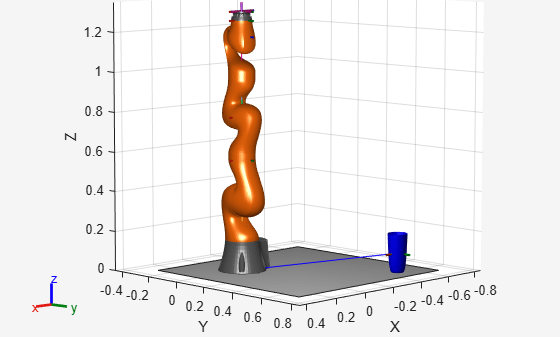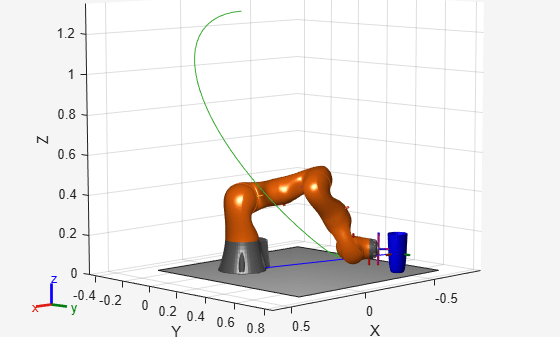# constraintCartesianBounds

Create constraint to keep body origin inside Cartesian bounds

## Description

The `constraintCartesianBounds` object describes a constraint on the position of one body (the end effector) relative to a target frame fixed on another body (the reference body). This constraint is satisfied if the position of the end-effector origin relative to the target frame remains within the `Bounds` specified. The `TargetTransform` property is the homogeneous transform that converts points in the target frame to points in the `ReferenceBody` frame.

Constraint objects are used in `generalizedInverseKinematics` objects to specify multiple kinematic constraints on a robot.

For an example that uses multiple constraint objects, see Plan a Reaching Trajectory With Multiple Kinematic Constraints.

## Creation

### Syntax

``cartConst = constraintCartesianBounds(endeffector)``
``cartConst = constraintCartesianBounds(endeffector,Name,Value)``

### Description

example

````cartConst = constraintCartesianBounds(endeffector)` returns a Cartesian bounds object that represents a constraint on the body of the robot model specified by `endeffector`.```
````cartConst = constraintCartesianBounds(endeffector,Name,Value)` returns a Cartesian bounds object with each specified property name set to the specified value by one or more `Name,Value` pair arguments. ```

### Input Arguments

expand all

End-effector name, specified as a string scalar or character vector. When using this constraint with `generalizedInverseKinematics`, the name must match a body specified in the robot model (`rigidBodyTree`).

Example: `"left_palm"`

Data Types: `char` | `string`

## Properties

expand all

Name of the end effector, specified as a string scalar or character vector. When using this constraint with `generalizedInverseKinematics`, the name must match a body specified in the robot model (`rigidBodyTree`).

Example: `"left_palm"`

Data Types: `char` | `string`

Name of the reference body frame, specified as a string scalar or character vector. The default `''` indicates that the constraint is relative to the base of the robot model. When using this constraint with `generalizedInverseKinematics`, the name must match a body specified in the robot model (`rigidBodyTree`).

Pose of the target frame relative to the reference body, specified as a matrix. The matrix is a homogeneous transform that specifies the relative transformation to convert a point in the target frame to the reference body frame.

Example: ```[1 0 0 1; 0 1 0 1; 0 0 1 1; 0 0 0 1]```

Bounds on end-effector position relative to target frame, specified as a 3-by-2 vector, ```[xMin xMax; yMin yMax; zMin zMax]```. Each row defines the minimum and maximum values for the xyz-coordinates respectively.

Weights of the constraint, specified as an ```[x y z]``` vector. Each element of the vector corresponds to the weight for the xyz-coordinates, respectively. These weights are used with the `Weights` property of all the constraints specified in `generalizedInverseKinematics` to properly balance each constraint.

## Examples

collapse all

This example shows how to use generalized inverse kinematics to plan a joint-space trajectory for a robotic manipulator. It combines multiple constraints to generate a trajectory that guides the gripper to a cup resting on a table. These constraints ensure that the gripper approaches the cup in a straight line and that the gripper remains at a safe distance from the table, without requiring the poses of the gripper to be determined in advance.

Set Up the Robot Model

This example uses a model of the KUKA LBR iiwa, a 7 degree-of-freedom robot manipulator. `importrobot` generates a `rigidBodyTree` model from a description stored in a Unified Robot Description Format (URDF) file.

```lbr = importrobot('iiwa14.urdf'); % 14 kg payload version lbr.DataFormat = 'row'; gripper = 'iiwa_link_ee_kuka';```

Define dimensions for the cup.

```cupHeight = 0.2; cupRadius = 0.05; cupPosition = [-0.5, 0.5, cupHeight/2];```

Add a fixed body to the robot model representing the center of the cup.

```body = rigidBody('cupFrame'); setFixedTransform(body.Joint, trvec2tform(cupPosition)) addBody(lbr, body, lbr.BaseName);```

Define the Planning Problem

The goal of this example is to generate a sequence of robot configurations that satisfy the following criteria:

• Start in the home configuration

• No abrupt changes in robot configuration

• Keep the gripper at least 5 cm above the "table" (z = 0)

• The gripper should be aligned with the cup as it approaches

• Finish with the gripper 5 cm from the center of the cup

This example utilizes constraint objects to generate robot configurations that satisfy these criteria. The generated trajectory consists of five configuration waypoints. The first waypoint, `q0`, is set as the home configuration. Pre-allocate the rest of the configurations in `qWaypoints` using `repmat`.

```numWaypoints = 5; q0 = homeConfiguration(lbr); qWaypoints = repmat(q0, numWaypoints, 1);```

Create a `generalizedInverseKinematics` solver that accepts the following constraint inputs:

• Cartesian bounds - Limits the height of the gripper

• A position target - Specifies the position of the cup relative to the gripper.

• An aiming constraint - Aligns the gripper with the cup axis

• An orientation target - Maintains a fixed orientation for the gripper while approaching the cup

• Joint position bounds - Limits the change in joint positions between waypoints.

```gik = generalizedInverseKinematics('RigidBodyTree', lbr, ... 'ConstraintInputs', {'cartesian','position','aiming','orientation','joint'})```
```gik = generalizedInverseKinematics with properties: NumConstraints: 5 ConstraintInputs: {1x5 cell} RigidBodyTree: [1x1 rigidBodyTree] SolverAlgorithm: 'BFGSGradientProjection' SolverParameters: [1x1 struct] ```

Create Constraint Objects

Create the constraint objects that are passed as inputs to the solver. These object contain the parameters needed for each constraint. Modify these parameters between calls to the solver as necessary.

Create a Cartesian bounds constraint that requires the gripper to be at least 5 cm above the table (negative z direction). All other values are given as `inf` or `-inf`.

```heightAboveTable = constraintCartesianBounds(gripper); heightAboveTable.Bounds = [-inf, inf; ... -inf, inf; ... 0.05, inf]```
```heightAboveTable = constraintCartesianBounds with properties: EndEffector: 'iiwa_link_ee_kuka' ReferenceBody: '' TargetTransform: [4x4 double] Bounds: [3x2 double] Weights: [1 1 1] ```

Create a constraint on the position of the cup relative to the gripper, with a tolerance of 5 mm.

```distanceFromCup = constraintPositionTarget('cupFrame'); distanceFromCup.ReferenceBody = gripper; distanceFromCup.PositionTolerance = 0.005```
```distanceFromCup = constraintPositionTarget with properties: EndEffector: 'cupFrame' ReferenceBody: 'iiwa_link_ee_kuka' TargetPosition: [0 0 0] PositionTolerance: 0.0050 Weights: 1 ```

Create an aiming constraint that requires the z-axis of the `iiwa_link_ee` frame to be approximately vertical, by placing the target far above the robot. The `iiwa_link_ee` frame is oriented such that this constraint aligns the gripper with the axis of the cup.

```alignWithCup = constraintAiming('iiwa_link_ee'); alignWithCup.TargetPoint = [0, 0, 100]```
```alignWithCup = constraintAiming with properties: EndEffector: 'iiwa_link_ee' ReferenceBody: '' TargetPoint: [0 0 100] AngularTolerance: 0 Weights: 1 ```

Create a joint position bounds constraint. Set the `Bounds` property of this constraint based on the previous configuration to limit the change in joint positions.

`limitJointChange = constraintJointBounds(lbr)`
```limitJointChange = constraintJointBounds with properties: Bounds: [7x2 double] Weights: [1 1 1 1 1 1 1] ```

Create an orientation constraint for the gripper with a tolerance of one degree. This constraint requires the orientation of the gripper to match the value specified by the `TargetOrientation` property. Use this constraint to fix the orientation of the gripper during the final approach to the cup.

```fixOrientation = constraintOrientationTarget(gripper); fixOrientation.OrientationTolerance = deg2rad(1)```
```fixOrientation = constraintOrientationTarget with properties: EndEffector: 'iiwa_link_ee_kuka' ReferenceBody: '' TargetOrientation: [1 0 0 0] OrientationTolerance: 0.0175 Weights: 1 ```

Find a Configuration That Points at the Cup

This configuration should place the gripper at a distance from the cup, so that the final approach can be made with the gripper properly aligned.

`intermediateDistance = 0.3;`

Constraint objects have a `Weights` property which determines how the solver treats conflicting constraints. Setting the weights of a constraint to zero disables the constraint. For this configuration, disable the joint position bounds and orientation constraint.

```limitJointChange.Weights = zeros(size(limitJointChange.Weights)); fixOrientation.Weights = 0;```

Set the target position for the cup in the gripper frame. The cup should lie on the z-axis of the gripper at the specified distance.

`distanceFromCup.TargetPosition = [0,0,intermediateDistance];`

Solve for the robot configuration that satisfies the input constraints using the `gik` solver. You must specify all the input constraints. Set that configuration as the second waypoint.

```[qWaypoints(2,:),solutionInfo] = gik(q0, heightAboveTable, ... distanceFromCup, alignWithCup, fixOrientation, ... limitJointChange);```

Find Configurations That Move Gripper to the Cup Along a Straight Line

Re-enable the joint position bound and orientation constraints.

```limitJointChange.Weights = ones(size(limitJointChange.Weights)); fixOrientation.Weights = 1;```

Disable the align-with-cup constraint, as the orientation constraint makes it redundant.

`alignWithCup.Weights = 0;`

Set the orientation constraint to hold the orientation based on the previous configuration (`qWaypoints(2,:)`). Get the transformation from the gripper to the base of the robot model. Convert the homogeneous transformation to a quaternion.

```fixOrientation.TargetOrientation = ... tform2quat(getTransform(lbr,qWaypoints(2,:),gripper));```

Define the distance between the cup and gripper for each waypoint

```finalDistanceFromCup = 0.05; distanceFromCupValues = linspace(intermediateDistance, finalDistanceFromCup, numWaypoints-1);```

Define the maximum allowed change in joint positions between each waypoint.

`maxJointChange = deg2rad(10);`

Call the solver for each remaining waypoint.

```for k = 3:numWaypoints % Update the target position. distanceFromCup.TargetPosition(3) = distanceFromCupValues(k-1); % Restrict the joint positions to lie close to their previous values. limitJointChange.Bounds = [qWaypoints(k-1,:)' - maxJointChange, ... qWaypoints(k-1,:)' + maxJointChange]; % Solve for a configuration and add it to the waypoints array. [qWaypoints(k,:),solutionInfo] = gik(qWaypoints(k-1,:), ... heightAboveTable, ... distanceFromCup, alignWithCup, ... fixOrientation, limitJointChange); end```

Visualize the Generated Trajectory

Interpolate between the waypoints to generate a smooth trajectory. Use `pchip` to avoid overshoots, which might violate the joint limits of the robot.

```framerate = 15; r = rateControl(framerate); tFinal = 10; tWaypoints = [0,linspace(tFinal/2,tFinal,size(qWaypoints,1)-1)]; numFrames = tFinal*framerate; qInterp = pchip(tWaypoints,qWaypoints',linspace(0,tFinal,numFrames))';```

Compute the gripper position for each interpolated configuration.

```gripperPosition = zeros(numFrames,3); for k = 1:numFrames gripperPosition(k,:) = tform2trvec(getTransform(lbr,qInterp(k,:), ... gripper)); end```

Show the robot in its initial configuration along with the table and cup

```figure; show(lbr, qWaypoints(1,:), 'PreservePlot', false); hold on exampleHelperPlotCupAndTable(cupHeight, cupRadius, cupPosition); p = plot3(gripperPosition(1,1), gripperPosition(1,2), gripperPosition(1,3));```Animate the manipulator and plot the gripper position.

```hold on for k = 1:size(qInterp,1) show(lbr, qInterp(k,:), 'PreservePlot', false); p.XData(k) = gripperPosition(k,1); p.YData(k) = gripperPosition(k,2); p.ZData(k) = gripperPosition(k,3); waitfor(r); end hold off```If you want to save the generated configurations to a MAT-file for later use, execute the following:

`>> save('lbr_trajectory.mat', 'tWaypoints', 'qWaypoints');`

## Version History

Introduced in R2017a

expand all

Behavior change in future release